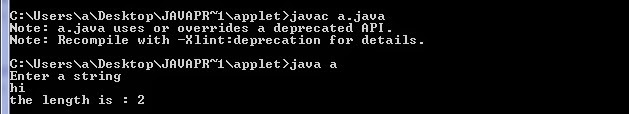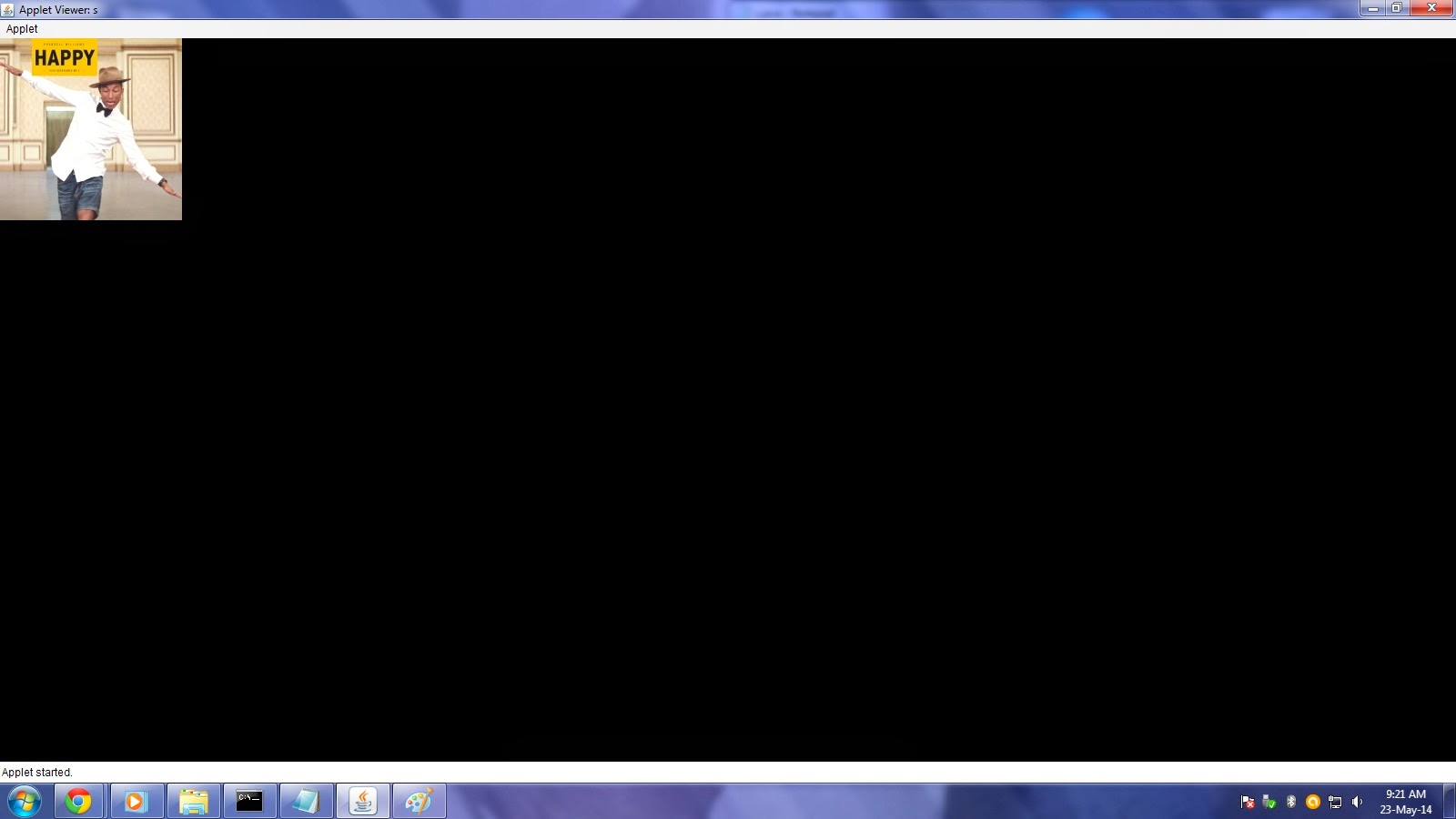## Posts

Showing posts from May 23, 2014

### Java 8 released

Are you still using jdk1.3
Java 8 is a revolutionary release of the world’s #1 development platform. It includes a huge upgrade to the Java programming model and a coordinated evolution of the JVM, Java language, and libraries. Java 8 includes features for productivity, ease of use, improved polyglot programming, security and improved performance. Welcome to the latest iteration of the largest, open, standards-based, community-driven platform.
source: http://www.oracle.com/technetwork/java/javase/overview/java8-2100321.html

### Length of the String without using java built in length methodimport java.io.*;
public class a
{
public static void main(String args[])throws Exception
{
String s ;
int i=0;

DataInputStream dd=new DataInputStream(System.in);
System.out.println("Enter a string");
s=s.trim();
try
{for(i=0;i>=0;i++)
s.charAt(i);
}
catch(Exception e)
{
System.out.println("the length is : "+i);
}

}
}

### count no. of words in java// count no. of words in java

import java.io.*;
public class a
{
public static void main(String args[])throws Exception
{
String s ;
int i,count=0;

DataInputStream dd=new DataInputStream(System.in);
System.out.println("Enter a string");
s=s.trim();
s=s+" ";
for(i=0;i<s.length();i++)
if(s.charAt(i)== ' ')
count+=1;
System.out.println("the no.of words are: "+count);

}

}

### Display alternate image in java applet// here we have used s1 and s2 images
import java.awt.*;
import java.awt.image.*;
import java.io.*;
import javax.imageio.*;
import java.applet.*;

import java.applet.Applet;

/*<applet code="s" height=678 width=678></applet>
*/
public class s extends Applet implements Runnable
{
int x=1;
String un="";
public void init()
{
t.start();
}
public void run()
{
try
{
while(true)
{
repaint();
t.sleep(500);

}
}catch(Exception e)
{
System.out.println(e);
}
}

public void update(Graphics g)
{
paint(g);
}
public void paint(Graphics g)
{

Dimension d=getSize();
g.fillRect(0,0,d.width,d.height);
try {
un="s"+x+".jpg";
x+=1;
if(x==3)
x=1;
}
catch (IOException e)
{
}
}
}

### pascal triangle

a=1 a=1
a=1
for i= 1 to n-1 { for j=1 to i-1 a[i]=1
a[i][i]= 1 }

for i = 0 to n -1 { for sp = n-1-i to 0 print a space

for j= 0 to j=i print a[i][j]

}

### Inserting image in java appletimport java.awt.*;
import java.awt.image.*;
import java.io.*;
import javax.imageio.*;

import java.applet.Applet;

/*<applet code="s" height=678 width=678></applet>
*/
public class s extends Applet
{

public void paint(Graphics g)
{
try {